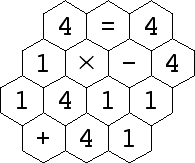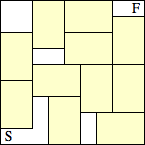2010 U. S. Championship Puzzles

1. Hex Equation

Find a path that passes through each hexagon exactly once, and traces out a true equation. The usual order of operations is used: multiplication and division before addition and subtraction.2. Rectangle Maze

Find a path that moves horizontally and vertically from the Start in the lower left to the Finish in the upper right. The path must pass through exactly two unit squares of each 2 × 3 rectangle.